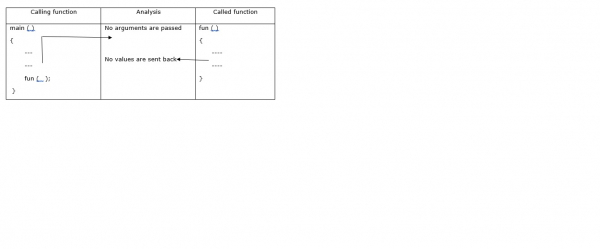# Explain the Character operations in C language

CServer Side ProgrammingProgramming

#### C in Depth: The Complete C Programming Guide for Beginners

45 Lectures 4.5 hours

#### Practical C++: Learn C++ Basics Step by Step

Most Popular

50 Lectures 4.5 hours

#### Master C and Embedded C Programming- Learn as you go

66 Lectures 5.5 hours

Character can be (A-Z(or) a- z), digit (0-9), a white space, or a special symbol in C programming language.

## Declaration

Following is the declaration for character operations in C programming −

char a= ‘A’; using a character constant.

## Character input / output functions

The character input/output functions are explained below −Example − char a;

scanf("%c", &a); printf ("%c", &a);
a = getchar ( ); putchar (a);
a = getch ( ); putch (a);

## Example

Following is the C program for line counting using getchar() −

Live Demo

#include <stdio.h>
/* count lines in input */
main(){
int count, num;
printf("enter multiple statements and Press cntrl+z:\n");
num = 0;
while ((count = getchar()) != EOF)
if (count == '\n')
++num;
printf("%d\n", num);
}

## Output

Following is the output of the program −

enter multiple statements and Press cntrl+z:
Hi
Hello
Welcome To
My World
^Z
4